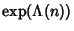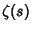## Mangoldt FunctionThe function defined by(1)is also given by [1, 2, ...,]/[1, 2, ...,], wheredenotes the Least Common Multiple. The first few values offor, 2, ..., plotted above, are 1, 2, 3, 2, 5, 1, 7, 2, ... (Sloane's A014963). The Mangoldt function is related to the Riemann Zeta Functionby(2)

where.The Summatory Mangoldt function, illustrated above, is defined by(3)

whereis the Mangoldt Function. This has the explicit formula(4)

where the second Sum is over all complex zerosof the Riemann Zeta Functionand interpreted as(5)

Vardi (1991, p. 155) also gives the interesting formula(6)

whereis the Nint function andis a Factorial.

Vallée Poussin's version of the Prime Number Theorem states that(7)

for some(Davenport 1980, Vardi 1991). The Riemann Hypothesis is equivalent to(8)

(Davenport 1980, p. 114; Vardi 1991).

See also Bombieri's Theorem, Greatest Prime Factor, Lambda Function, Least Common Multiple, Least Prime Factor, Riemann Function

References

Davenport, H. Multiplicative Number Theory, 2nd ed. New York: Springer-Verlag, p. 110, 1980.

Sloane, N. J. A. Sequence A014963 in The On-Line Version of the Encyclopedia of Integer Sequences.'' http://www.research.att.com/~njas/sequences/eisonline.html.

Vardi, I. Computational Recreations in Mathematica. Reading, MA: Addison-Wesley, pp. 146-147, 152-153, and 249, 1991.